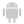Home |Sign in | EnglishHelpWLanguageWLanguage functionsControls, pages and windowsDrawing functionsPrefix syntaxDrawing functions on WDPic variablesDrawChordPresentationExampleLimitsCoordinatesSolid figureRelated examplesSee alsoOpenSaveAlphaBlendBackgroundChangeModeDrawArcDrawChordDrawCircleDrawLineDrawPointDrawPolygonDrawPolylineDrawRectangleDrawRectangleGradientDrawRoundedRectangleDrawSliceDrawTextDrawTextRTFEndDrawingFillFontHorizontalSymmetryInvertColorMergeLayerPixelColorPixelOpacityResizeRotationSaveStartDrawingVerticalSymmetryPicMergeLayerPicOpenPicResizePicRotationPicSave
• Limits
• Coordinates
• Solid figure
WINDEVWEBDEVWINDEV MobileOthers<WDPic variable>.DrawChord (Function)
In french: <Variable WDPic>.DessineCorde
Draws the chord of a circle (intersection between an ellipse and a straight line) in a WDPic variable.
Example
MonImage est un WDPic = "Test.gif"
// Dessin dans une variable WDPic d'une corde de cercle dont le
// fond est jaune clair et le bord bleu clair
MonImage.DessineCorde(10, 10, 50, 50, 20, 20, 40, 50, JauneClair, BleuClair)

IMG_MonDessin = MonImage
Syntax
<WDPic image>.DrawChord(<X1> , <Y1> , <X2> , <Y2> , <X3> , <Y3> , <X4> , <Y4> [, <Background color> [, <Line color>]])
<WDPic image>: WDPic variable
Name of the variable of type WDPic to be used. Only the background layer will be handled.
<X1>: Integer
X-coordinate of top left corner of the rectangle containing the circle. These coordinates are expressed in pixels.
<Y1>: Integer
Y-coordinate of top left corner of the rectangle containing the circle. These coordinates are expressed in pixels.
<X2>: Integer
X-coordinate of bottom right corner of the rectangle containing the circle. These coordinates are expressed in pixels.
<Y2>: Integer
Y-coordinate of bottom right corner of the rectangle containing the circle. These coordinates are expressed in pixels.
<X3>: Integer
X-coordinate of start point of the chord. These coordinates are expressed in pixels.
<Y3>: Integer
Y-coordinate of start point of the chord. These coordinates are expressed in pixels.
<X4>: Integer
X-coordinate of end point of the chord. These coordinates are expressed in pixels.
<Y4>: Integer
Y-coordinate of end point of the chord. These coordinates are expressed in pixels.
<Background color>: Integer or constant (optional)
Background color of chord. This color can correspond to:
If this parameter is not specified, the background color:
<Line color>: Integer or constant (optional)
Line color for the circle chord. This color can correspond to:
If this parameter is not specified, the line color:
Remarks

Limits

• The <WDPic variable>.DrawChord function is not available in Anti-aliasing mode (see the <WDPic variable>.ChangeMode function help for details).
• <WDPic variable>.DrawChord is not available for a drawing with management of alpha channel (see the help about <WDPic variable>.StartDrawing for more details). This function has no effect.

Coordinates

If the start and end points are not located on the outline of the circle (or on the outline of the ellipse), the point taken into account is the intersection between the circle and the line that joins the specified point and the center of the rectangle (which means the center of the circle).Coordinates are indicated relative to the top left corner of the Image control (coordinates (0.0)).

Solid figure

<WDPic variable>.DrawChord can only be used to draw a chord: outline only or chord area. To draw a solid figure (portion defined by the center of the circle and the two points on the circle), use <WDPic variable>.DrawSlice.
Related Examples:Unit examples (WINDEV): The drawing functions [ + ] Using the main drawing functions of WINDEV to:- Initialize an Image control for drawing- Draw simple shapes- Write a text into a drawing- Change the color in a drawing
Business / UI classification: Neutral code
Component: wd270pnt.dll
Minimum version required
• Version 25